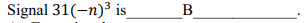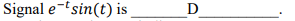# Linear Systems Analysis And Design

1. A. Even signal
B. Odd signal
C. Neither even nor odd signal
D. Both even and odd signal
2.A. Even signal
B. Odd signal
C. Neither even nor odd signal
D. Both even and odd signal
3. Signal 𝑥 = cos (202101𝜋𝑡) is ______A_____________.
A. Even signal
B. Odd signal
C. Neither even nor odd signal
D. Both even and odd signal
4.A. Even signal
B. Odd signal
C. Neither even nor odd signal
D. Both even and odd signal
5. Which of the following statements regarding signals is false? B
A. f (t) is a periodic signal if f (t) = f (t + mT ), for any integers m .
B. Even signals are antisymmetric about the vertical axis or time origin.
C. A discrete-time signal can be derived from a continuous-time signal by sampling.
D. There is no uncertainty with respect to the value of a deterministic signal at any time
6. Signal 𝑠𝑖𝑛(3𝑛)/𝑐𝑜𝑠(−3𝑛) is ______B_____________.
A. Discrete-time periodic
B. Discrete-time aperiodic
C. Continuous-time periodic
D. Continuous-time aperiodic
7. Signal 𝑐𝑜𝑠(2𝜋 − 5𝜋𝑛/3) is ______A____________.
A. Discrete-time periodic
B. Discrete-time aperiodic
C. Continuous-time periodic
D. Continuous-time aperiodic
8.A. Discrete-time periodic
B. Discrete-time aperiodic
C. Continuous-time periodic
D. Continuous-time aperiodic
9. Signal 𝑢[𝑛 + 1] is _______A___________.
A. A power signal
B. An energy signal
C. Both power and energy signal
D. Neither power or energy signal
10.A. Discrete-time periodic
B. Discrete-time aperiodic
C. Continuous-time periodic
D. Continuous-time aperiodic
11. Which of the following statements given below is true? A
A. A signal cannot be a power signal as well as an energy signal.
B. A signal can be a power signal as well as an energy signal.
C. If a signal has an instant power of 10W, it is a power signal.
D. If a signal has a total energy of 0.00001 joules, it is not an energy signal
12. If signal 𝑦(𝑡) = 𝑥(0.5𝑡), then the amplitude of signal 𝑦(𝑡) is B
A. twice that of 𝑥(𝑡).
B. the same as 𝑥(𝑡).
C. half that of 𝑥(𝑡).
D. amplitude of 𝑥(𝑡) decreased by value 2
13. Signal 𝑥(3𝑡) is signal 𝑥(𝑡) ___C_____ by a factor of 3.
A. amplified
B. attenuated
C. time-compressed
D. time-expanded